（给ImportNew加星标，提高Java技能）

### 一、斐波那契数列

1、暴力递归 **

``````int fib(int N) {
if (N == 1 || N == 2) return 1;
return fib(N - 1) + fib(N - 2);
}

``````

PS：但凡遇到需要递归的问题，最好都画出递归树，这对你分析算法的复杂度，寻找算法低效的原因都有巨大帮助。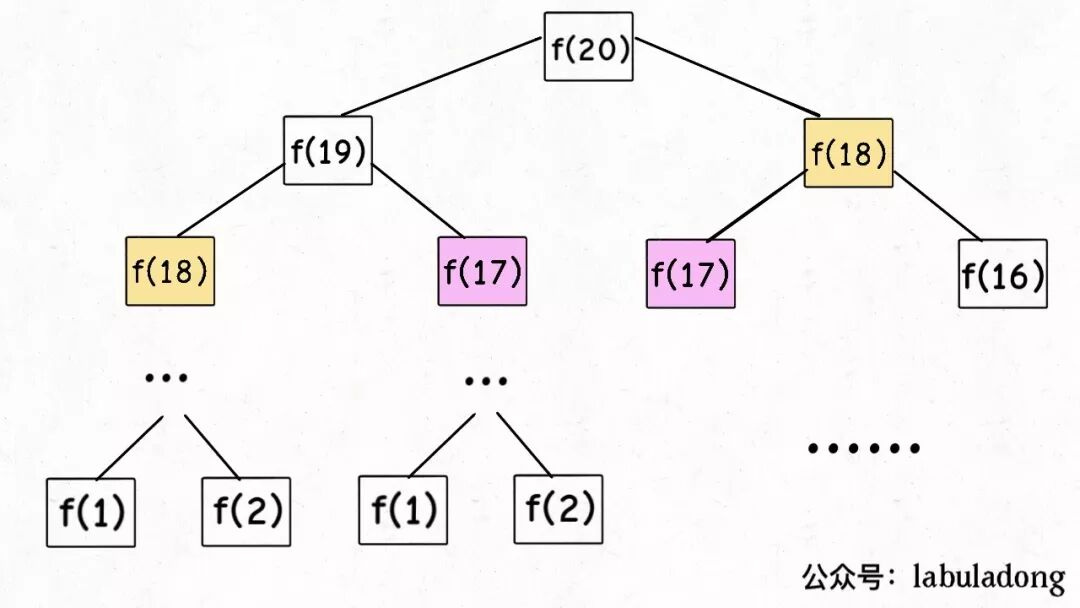2、带备忘录的递归解法 **

``````int fib(int N) {
if (N < 1) return 0;
// 备忘录全初始化为 0
vector<int> memo(N + 1, 0);
// 初始化最简情况
return helper(memo, N);
}

int helper(vector<int>& memo, int n) {
// base case
if (n == 1 || n == 2) return 1;
// 已经计算过
if (memo[n] != 0) return memo[n];
memo[n] = helper(memo, n - 1) +
helper(memo, n - 2);
return memo[n];
}

``````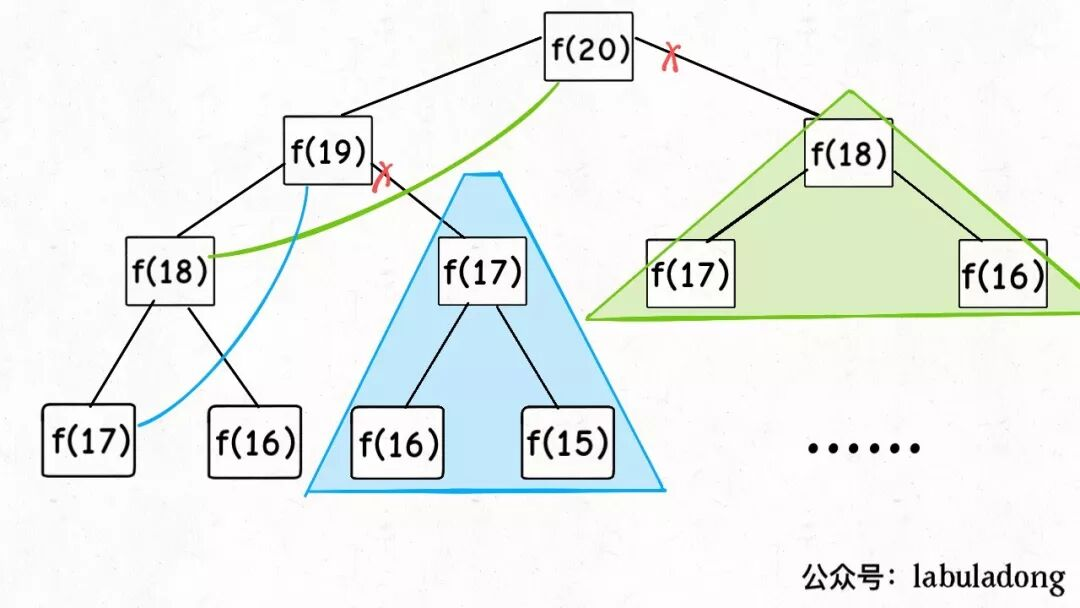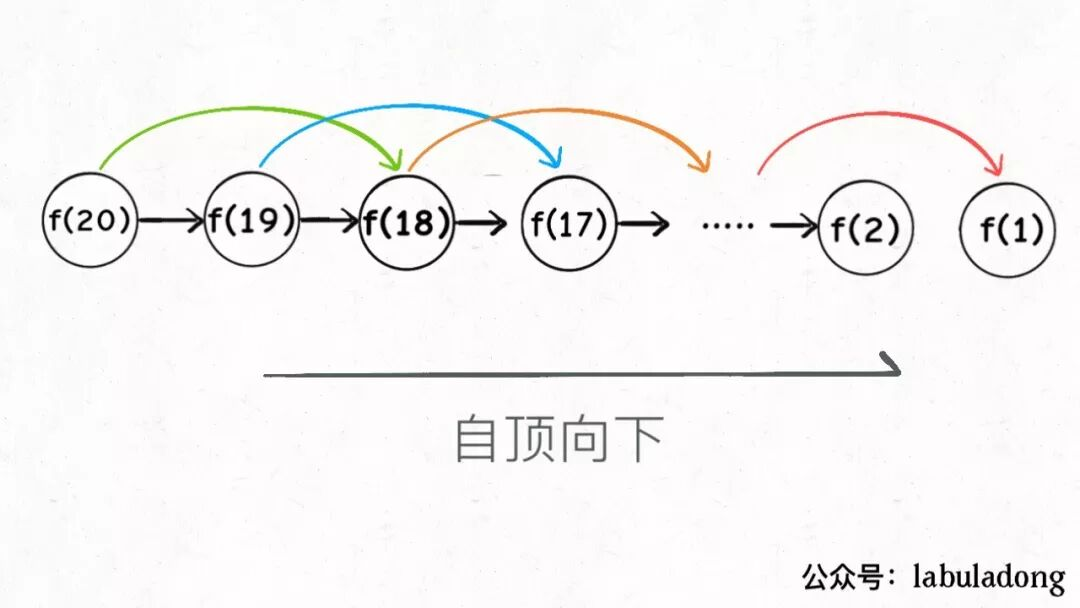3、dp 数组的迭代解法 **

``````int fib(int N) {
vector<int> dp(N + 1, 0);
// base case
dp = dp = 1;
for (int i = 3; i <= N; i++)
dp[i] = dp[i - 1] + dp[i - 2];
return dp[N];
}

``````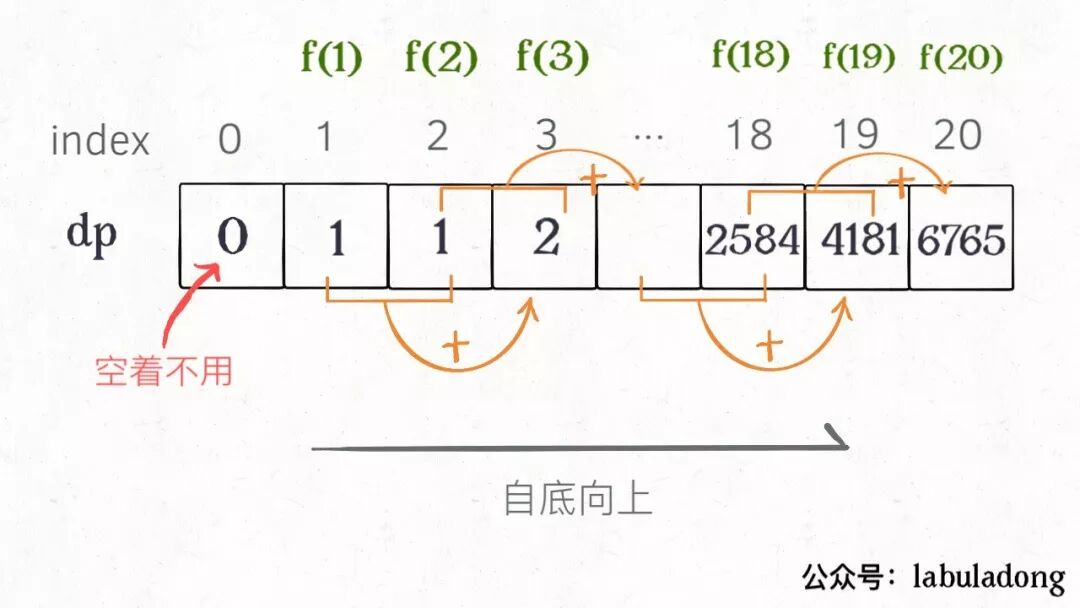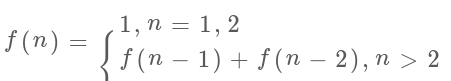``````int fib(int n) {
if (n == 2 || n == 1)
return 1;
int prev = 1, curr = 1;
for (int i = 3; i <= n; i++) {
int sum = prev + curr;
prev = curr;
curr = sum;
}
return curr;
}

``````

### 二、凑零钱问题

``````// coins 中是可选硬币面值，amount 是目标金额
int coinChange(int[] coins, int amount);

``````

1、暴力递归 **

``````# 伪码框架
def coinChange(coins: List[int], amount: int):
# 定义：要凑出金额 n，至少要 dp(n) 个硬币
def dp(n):
# 做选择，需要硬币最少的那个结果就是答案
for coin in coins:
res = min(res, 1 + dp(n - coin))
return res
# 我们要求目标金额是 amount
return dp(amount)

``````

``````def coinChange(coins: List[int], amount: int):

def dp(n):
# base case
if n == 0: return 0
if n < 0: return -1
# 求最小值，所以初始化为正无穷
res = float('INF')
for coin in coins:
subproblem = dp(n - coin)
# 子问题无解，跳过
if subproblem == -1: continue
res = min(res, 1 + subproblem)

return res if res != float('INF') else -1

return dp(amount)

``````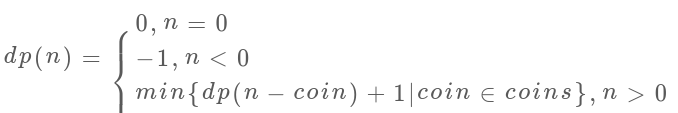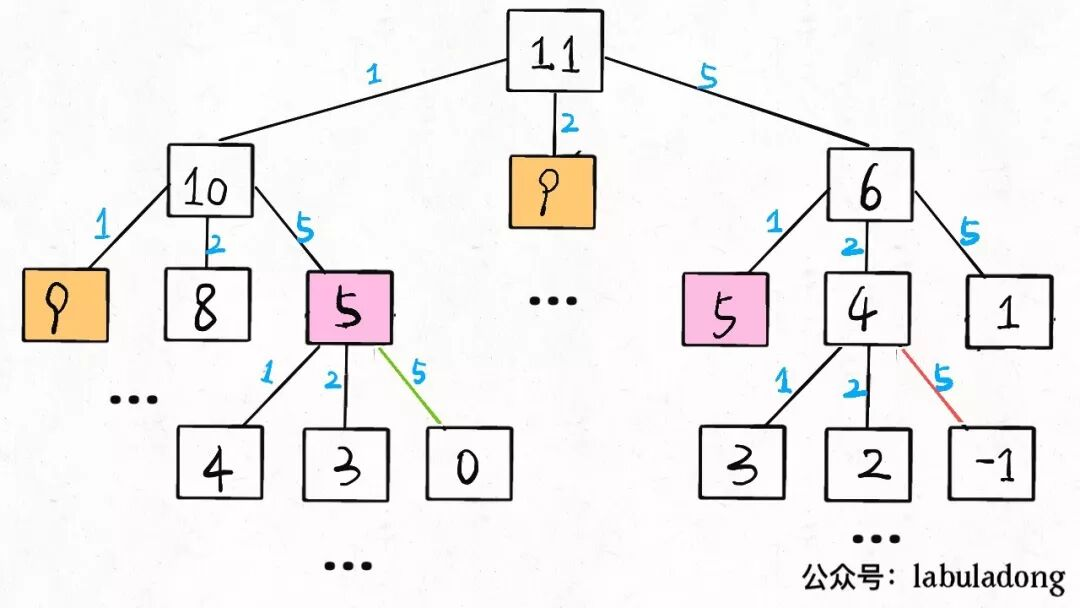2、带备忘录的递归 **

``````def coinChange(coins: List[int], amount: int):
# 备忘录
memo = dict()
def dp(n):
# 查备忘录，避免重复计算
if n in memo: return memo[n]

if n == 0: return 0
if n < 0: return -1
res = float('INF')
for coin in coins:
subproblem = dp(n - coin)
if subproblem == -1: continue
res = min(res, 1 + subproblem)

# 记入备忘录
memo[n] = res if res != float('INF') else -1
return memo[n]

return dp(amount)

``````

3、dp 数组的迭代解法 **

** `dp[i] = x` 表示，当目标金额为 `i` 时，至少需要 `x` 枚硬币** 。

``````int coinChange(vector<int>& coins, int amount) {
// 数组大小为 amount + 1，初始值也为 amount + 1
vector<int> dp(amount + 1, amount + 1);
// base case
dp = 0;
for (int i = 0; i < dp.size(); i++) {
// 内层 for 在求所有子问题 + 1 的最小值
for (int coin : coins) {
// 子问题无解，跳过
if (i - coin < 0) continue;
dp[i] = min(dp[i], 1 + dp[i - coin]);
}
}
return (dp[amount] == amount + 1) ? -1 : dp[amount];
}

``````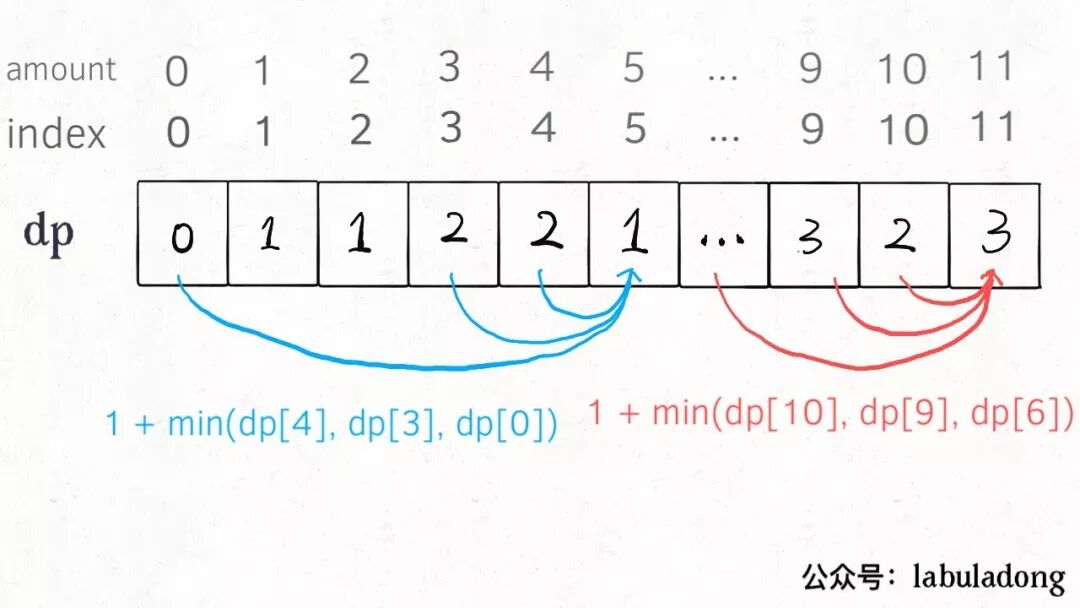PS：为啥 `dp` 数组初始化为 `amount + 1` 呢，因为凑成 `amount` 金额的硬币数最多只可能等于 `amount` （全用 1 元面值的硬币），所以初始化为 `amount + 1` 就相当于初始化为正无穷，便于后续取最小值。

### 三、最后总结